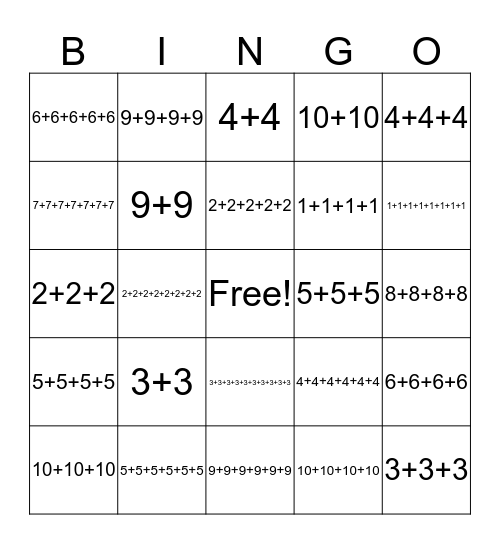This bingo card has a free space and 24 words: 3+3, 5+5+5+5+5+5, 2+2+2+2+2, 10+10+10, 4+4+4, 6+6+6+6+6, 7+7+7+7+7+7+7, 9+9+9+9, 5+5+5, 3+3+3+3+3+3+3+3+3+3, 1+1+1+1, 2+2+2, 4+4+4+4+4+4, 6+6+6+6, 9+9, 10+10+10+10, 8+8+8+8, 4+4, 1+1+1+1+1+1+1+1, 2+2+2+2+2+2+2+2, 3+3+3, 5+5+5+5, 10+10 and 9+9+9+9+9+9.

## Play Online